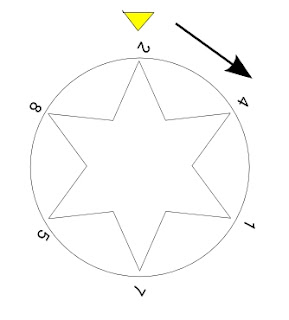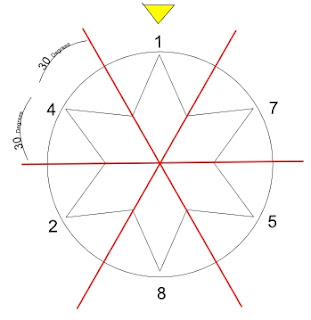## Friday, March 13, 2009

### 6 Days of Creation - the Ring, the Sphere, and Jimi Hendrix

But this is an excellent one for a Friday the 13th!
Love and Unity to you all.
(and besides tomorrow is 3/14, my day for pie!)

Let me introduce you to...

(and Let me preface all this by telling you that I'm not Jewish)

and also a very basic knowledge of Zohar & GenIsis/OT/Torah.
(though this post is applicable to MORE than Zohar, OT and Genesis - any Physicists in the readership may find even more to it!)

Looking at the very first word of Genesis,
BeReSHiTH

which can become
BeReSHiTH to BaRa-SHiTH

BARA-SHITH
which means

Now we consider our ABC's - the letters/characters
22 characters of the Hebrew Alephbet
7 days of Creation (6 +1 OF REST)

22 / 7 = 3.142857142857142857142857142857142857...

and that's how REAL men bake Pi π
(LOL)

We can see in that ancient pi π a repeating sequence of:

"142857"

and now to the "BOOK"

In the Beginning was the Word...
and the void...

142857 x 0 = 0

142857 x 1 = 142857
142857 x 2 = 285714
142857 x 3 = 428571
142857 x 4 = 571428
142857 x 5 = 714285
142857 x 6 = 857142

on the Seventh day he rested as his work was complete...

142857 x 7 = 999999

(You'll note there's SIX nines there)

Let's explore some more curiosities of this wonderful number 142857:

1 + 4 + 2 + 8 + 5 + 7 = 27 = 9

142 + 857 = 999

14 + 28 + 57 = 99

1 4 2
8 5 7
- - -
9 9 9

The Number 9, - the final of all the single digits.

0, 1, 2, 3, 4, 5, 6, 7, 8, 9

Now we turn our thought to 3 dimensions

and imagine these numbers around the surface of a sphere or better yet a ring

we know it is these figures derived from the 2 dimensional
circle because the original calculation gave us PI (22/7)

(We will also find the formation of Magen DaViD)
(I'll add some graphics to illustrate the 3D and rings later)

(Synchromystics will recognize the 15, 24, 33, 42, 51 appearing)

Now we can imagine we are holding our ring(s) with the 142857 sequence on it at eye level...

Or even something like this:142857 x 1 = 142857

2 character places turned forward (clockwise)...
OR
4 character places turned back (counter clockwise)...

(2+4 = 6)142857 x 2 = 285714

5 character places turned forward...
OR
1 character places turned back...

(5+1 = 6)

142857 x 3 = 428571

3 character places turned forward...
OR
3 character places turned back...

(3+3 = 6)

142857 x 4 = 571428

1 character places turned forward...
OR
5 character places turned back...

(1+5 = 6)

142857 x 5 = 714285

4 character places turned forward...
OR
2 character places turned back...

(2+4 = 6)

142857 x 6 = 857142

We find the cycling of the

2+4
1+5
3+3
5+1
4+2

and at seven it all changes so we've come full circle and we rest.

The digit 6 rotated clockwise or counter-clockwise by 180 degrees (6 x 30) gives us the digit 9

6 9

Now why did I bring up 6 and 9
Aside from the Six Nines at the 7th turn/day!

6th turn and 8+1
8 in the "1"'s position (where the "1" was when we first began our turning)
8+1 = 9

(Our little number ring is 180 degrees from where we started!)Here's the CYCLING of 142857 that we witnessed while turning ...

142857 x 1 = 142857
142857 x 2 = 285714
142857 x 3 = 428571
142857 x 4 = 571428
142857 x 5 = 714285
142857 x 6 = 857142

142857 x 7 = 999999 (the 7th turn = the 7th day meets completion in the all "9" result which is the final of the 0-9 digits)6 x 30 = 180
(6 x 60 = 360)

and J H's "If 6 was 9" plays in the background...
(JH JaH, IeH)

9 = NINE
N+I+N+E = 42
9 = 4+2 = 6

If '6' was '9'

Much more to come on this topic in future posts.
But for now, we can enjoy Jimi's tunez...

And let me introduce you to the first MAN / AMN
otherwise known as ADAM / ATOM“If you only knew the magnificence of the 3, 6 and 9, then you would have the Key to the UNIVERSE.”
- Nikola Telsa

The Enneagram:Image courtesy of KA Gold JeweleryUnknown said...

Excellent, Strangie.

Gives me something to think about.

Andre
The ALIEN Project
www.thealienproject.blogspot.com

mike thomas said...

I see it icy it ycy it

Not sure if you quite got my drift re last post, but what the hell.

Lots og eegipshun stuff on your site, you must be a disciple of the great Rah.

By the way, you seem very into this blogging stuff, and I wondered if you might know of a good site for beginners like me?

regardsAnonymous said...

So you don't spend any more time musing over the wrong number,

22/7 is an approximation of PI. PI is the number that you get when you divide the circumference of a circle by its radius & reduce this number, for all perfect 2D circles. PI is irrational, which is to say that it has an infinite number of decimal values with no repeating pattern.

Given radius r is any number and
circumference c = 2PIr,

c/r = (2PIr)/r = 2PI

Its always the same number for all r, 3.141592659... etc, forever.

A more interesting constant is that all electrons have the exact same mass.

Here is some really good shit:

StrangEye said...

George,

you have the right to believe whatever you want.

Unfortunately the modern PI (which IN FACT is not constant and is most definitely DISPUTED by the leading multi-doctrinal mathematicians of the world) does not unlock the mysteries encoded in the texts whereas 22/7 does.

You can believe what want, but believing instead of knowing was how the truth was lost to the masses in the first place.

Cheers,

~SE~Anonymous said...

yeah that is a wild number sequence ... it would make a good tattooAnonymous said...

see I should tattoo it to myself so I don't forget

StrangEye said...

Something tells me you won't!!

This number, and the resultant Enneagram figure, are haunting in the power of their synchs!

~SE~